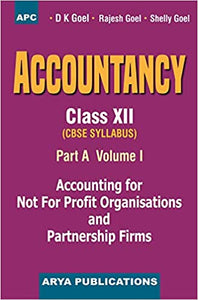# Accountancy (Part-A) Vol-I, Class- XII 2020

Regular price
Rs. 482.62
Sale price
Rs. 482.62
Regular price
Rs. 495.00
Sold out
Unit price
per

Some of the special features of the book are :

The book contains True-False, Fill in the Blanks, matching, Multiple Choice, Short-answer type and practical questions at the end of each chapter so that students may test their understanding of the chapter.

Large number of questions based on Incomplete Information (i.e., missing figure questions) have been given at the end of every chapter. A number of illustrations have been given in each chapter and these have been solved in such a simple manner that students can easily understand them. Practical questions given at the end of each chapter are based on latest CBSE question papers and these are numbered according to the illustrations. Answers and hints to solve the questions have been given at the end of each question. We are very much confident that after doing the illustrations, students can independently solve the practical questions.

Weightage to each topic has been given according to the marks allotted to it by the CBSE.

Accuracy is one the main features of the book.

Part A is bifurcated in two Volumes for the convenience of the students. Volume I Comprises of Accounting for Not-for-Profit Organisations and Partnership Firms whereas Volume II has Company Accounts.